# JP2005269807A - Switching regulator - Google Patents

## Info

Publication number
JP2005269807A
JP2005269807A JP2004080327A JP2004080327A JP2005269807A JP 2005269807 A JP2005269807 A JP 2005269807A JP 2004080327 A JP2004080327 A JP 2004080327A JP 2004080327 A JP2004080327 A JP 2004080327A JP 2005269807 A JP2005269807 A JP 2005269807A
Authority
JP
Japan
Prior art keywords
voltage
circuit
output voltage
capacitor
output
Prior art date
Legal status (The legal status is an assumption and is not a legal conclusion. Google has not performed a legal analysis and makes no representation as to the accuracy of the status listed.)
Pending
Application number
JP2004080327A
Other languages
Japanese (ja)
Inventor
Ryotaro Kudo

Original Assignee
Renesas Technology Corp

Priority date (The priority date is an assumption and is not a legal conclusion. Google has not performed a legal analysis and makes no representation as to the accuracy of the date listed.)
Filing date
Publication date
Application filed by Renesas Technology Corp, 株式会社ルネサステクノロジ filed Critical Renesas Technology Corp
Priority to JP2004080327A priority Critical patent/JP2005269807A/en
Publication of JP2005269807A publication Critical patent/JP2005269807A/en
Application status is Pending legal-status Critical

## Images

••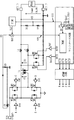•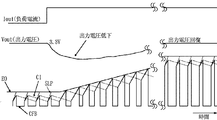•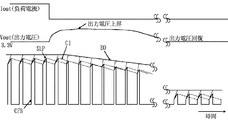•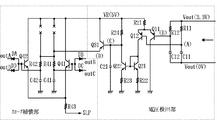•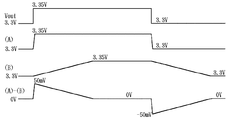•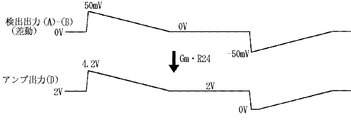•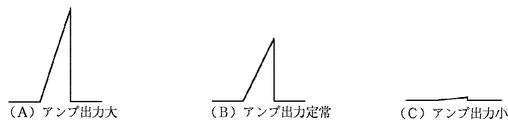•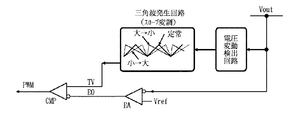••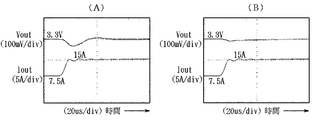•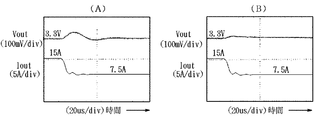•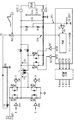•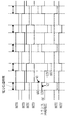•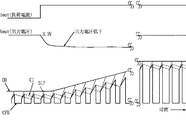•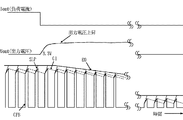## Abstract

PROBLEM TO BE SOLVED: To provide a switching power supply having both low loss and both stability and responsiveness.
An AC voltage formed by an inductive load is smoothed by a smoothing circuit to form a DC output voltage, which is input to an error amplifier that receives a reference voltage, the output voltage and a current component that flows through the inductive load, and A slope compensation component is input to a PWM generation circuit to form a PWM signal such that the DC output voltage becomes a desired voltage corresponding to the reference voltage, and the inductive load is driven via a drive circuit. Based on the voltage fluctuation detection of the output voltage, the slope compensation component is modulated so as to increase or decrease the slope compensation component in response to a change in the positive or negative direction of the output voltage.
[Selection] Figure 1

## Description

The present invention relates to a switching power supply, for example, a technique effective when applied to a switching power supply that constitutes an isolated DC / DC converter that converts a high voltage into a low voltage.

FIG. 13 shows a block diagram of an isolated DC / DC converter having a + 48V input and a 3.3V output, which was examined prior to the present invention. In this DC / DC converter, the output voltage V2 of the main transformer T1 is full-wave rectified by the choke coils L1 and L2 and the capacitor CB to form the output voltage Vout. The feedback voltage VFB obtained by dividing the output voltage Vout by the voltage dividing resistors R1 and R2 and the reference voltage Vref are input to the error amplifier EA to form a differential voltage. The output voltage of the error amplifier EA and the voltage obtained by adding the slope compensation signal SLP to the signal CV corresponding to the current slope obtained by extracting the current flowing through the main transformer T1 by the current transformer CT are input to the PWM modulation circuit PWMG, and A PWM signal is formed such that the feedback voltage VFB and the reference voltage Vref match. As shown in FIG. 14, input signals A to D and E, F of the driving circuit of the transformer T1 are formed by this PWM signal. Thus, the time for driving the transformer T1 (power transmission time) is determined. This power transmission period corresponds to the pulse width of the PWM signal. Examples of the DC / DC converter using the slope compensation signal include Japanese Patent Laid-Open Nos. 2001-112250 and 2003-284339.
JP 2001-112250 A JP 2003-284339 A

In the DC / DC converter as described above, the load response characteristic is determined by the response speed of the error amplifier EA. Therefore, when there is a high load current fluctuation (High di / dt), the power supply output decreases (or increases). There is a problem of being big. In addition, the time until returning to the steady voltage also becomes longer. For example, as shown in FIG. 15, the transient response of the load current small → large changes the output EO of the error amplifier EA depending on the speed, and the slope compensation signal SLP is applied to the signal CV corresponding to the current flowing through the main transformer. A PWM signal is formed so that the power period ends when the applied voltage reaches, and the current of the main transformer T1 increases following the output EO of the error amplifier EA. In the process in which the current of the main transformer T1 increases, the time for applying the voltage to the main transformer T1 is gradually increased to reach the current level corresponding to the target output current value.

On the contrary, as shown in FIG. 16, the load current large → small transient response causes the output EO of the error amplifier EA to change depending on its speed, and the current of the main transformer T1 decreases following the change as described above. To go. In the process of decreasing the current of the main transformer T1, the time for applying the voltage to the transformer T1 is gradually shortened to a current level corresponding to the target output current value. In FIG. 15 and FIG. 16, the dotted line indicates the current flowing through the secondary choke coil L1 or L2 of the main transformer T1. Therefore, it is sufficient to increase the response speed of the error amplifier EA, but high speed operation is possible at the time of load fluctuation as described above, but the error amplifier EA responds excessively at the time of steady operation with little load fluctuation, There is a problem that the stability of the output voltage Vout is deteriorated. That is, in the conventional DC / DC converter, the stability and the responsiveness are in a contradictory relationship, and the circuit setting is generally performed so as to sacrifice the responsiveness. Such a circuit setting is called phase compensation, and the circuit is called a phase compensation circuit.

An object of the present invention is to provide a switching power supply that achieves both stability and responsiveness. Another object of the present invention is to provide a switching power supply that achieves both stability and responsiveness with low loss. The above and other objects and novel features of the present invention will be apparent from the description of this specification and the accompanying drawings.

The outline of a typical invention among the inventions disclosed in the present application will be briefly described as follows. That is, an AC voltage formed by an inductive load is smoothed by a smoothing circuit to form a DC output voltage, which is input to an error amplifier that receives a reference voltage. The output voltage, the current component flowing through the inductive load, and slope compensation Component is input to the PWM generation circuit, a PWM signal is formed so that the DC output voltage becomes a desired voltage corresponding to the reference voltage, the inductive load is driven through the drive circuit, and the slope compensation is performed. Based on the voltage fluctuation detection of the output voltage, the component is modulated so as to increase or decrease the slope compensation component in response to a change in the positive or negative direction of the output voltage.

A switching power supply that achieves both stability and responsiveness with low loss can be realized.

FIG. 2 is a schematic block diagram showing an embodiment of the switching power supply according to the present invention. In the basic configuration of this DC / DC converter, the output voltage V2 of the main transformer T1 is full-wave rectified by the choke coils L1, L2 and the capacitor CB in the same manner as the DC / DC converter of FIG. The feedback voltage VFB obtained by dividing the output voltage Vout by the voltage dividing resistors R1 and R2 and the reference voltage Vref are input to the error amplifier EA phase-compensated by the phase compensation circuit to form a differential voltage. The slope compensation signal SLP formed by the slope compensation circuit SLMC according to the present invention is added to the signal CV corresponding to the current slope obtained by the output voltage EO of the error amplifier EA and the current flowing through the main transformer T1 by the current transformer CT. Is input to the PWM modulation circuit PWMG as a current feedback signal CFB to form a PWM signal in which the feedback voltage VFB and the reference voltage Vref coincide with each other. As shown in FIG. The input signals A to D and E, F of the drive circuit of the transformer T1 are formed to determine the time (power transmission time) for driving the main transformer T1. FIG. 1 shows an example of the slope compensation circuit SLMC. An example block diagram is shown. The output voltage Vout is transmitted to the error amplifier EA and the voltage fluctuation detection circuit of the slope compensation circuit SLMC as the voltage feedback signal VFB through the voltage dividing circuit as described above. In the voltage fluctuation detection circuit, a signal in which the fluctuation of the output voltage Vout is detected is transmitted to the slope modulation circuit. In the slope modulation circuit, a slope compensation signal SLP modulated corresponding to the output signal of the voltage fluctuation detection circuit is formed. The slope compensation signal SLP and the current slope signal CV are combined (added) by an adder circuit ADD to generate the current feedback signal CFB. The PWM comparator CMP receives the current feedback signal CFB and the output signal EO of the error amplifier EA to form a PWM signal. The control logic circuit included in the control circuit of FIG. Such drive signals A to F of the main transformer T1 are formed.

The slope compensation signal SLP is switched according to the input voltage level between the output signal EO and the current feedback signal CFB by the comparator CMP for generating the PWM signal when the output signal EO of the error amplifier EA and the current feedback signal CFB are input. In order to prevent the PWM signal from becoming unstable due to a slight fluctuation in noise, etc., this is a change signal whose value changes with time in order to give the current feedback signal a time slope. .

The drive signals A to D are supplied to the drive circuits DVA to DVD, and perform on / off control of the switch MOSFETs M1 to M4 constituting the bridge circuit. The bridge circuit is composed of N-channel type power switch MOSFETs M1, M2 and M3, M4 provided in series between the input voltage VIN (+ 48V) and the ground potential. An input side terminal of the main transformer T1 is connected to a connection point between the MOSFETs M1 and M2 and a connection point between the MOSFETs M3 and M4. Further, the ground potential of the circuit is applied to both ends on the output side of the main transformer T1 through the switch MOSFETs M5 and M6. The drive circuits DVE and DVF receive the signals E and F and drive the MOSFETs M5 and M6, respectively.

As shown in the timing diagram of FIG. 14, the signals A and B and C and D change complementarily. When the signal A is at a high level and the signal D is at a high level, a current flows through the path of the switch MOSFET M1-transformer T1-switch MOSFET M4, the MOSFET M6 is turned on by the high level of the signal F, and one of the output sides of the transformer T1. Is given a ground potential to form a positive voltage. During a period in which the signal B is at a high level and the signal C is at a high level, a current flows through the path of the switch MOSFET M3-transformer T1-switch MOSFET M2, the MOSFET M5 is turned on by the high level of the signal E, and the other on the output side of the transformer T1 Is given a ground potential to form a positive voltage. Thus, a stepped-down full-wave output is formed with respect to the positive / negative input inputted to the transformer T1, and this is rectified by the choke coil L1 or L2 and the condenser CB to form the output voltage Vout.

FIG. 3 is a waveform diagram for explaining an example of the operation of the switching power supply according to the present invention. In the figure, an example of the transient response when the load current Iout changes from small to large is shown. Basically, as described above, the output signal EO of the error amplifier EA changes depending on its speed, and the current of the main transformer T1 increases accordingly. In the process in which the current of the main transformer T1 increases, the time during which the voltage V1 is applied to the transformer is gradually increased to a current level corresponding to the target output current value. In this embodiment, a period during which the output voltage Vout fluctuates (output voltage drop) is detected by the voltage fluctuation detection circuit, and the slope compensation signal SLP is modulated so as to be extremely small (zero in the figure) during that period. As a result, the time during which the voltage V1 is applied to the main transformer T1 becomes longer.

When the slope compensation signal SLP is made extremely small as in this embodiment, the current slope signal CV becomes the current feedback signal CFB, and the output of the comparator CMP is not inverted until the error amplifier output EO is reached, so the pulse width of the PWM signal is long. Thus, the power transmission period is lengthened. This makes it possible to increase the current CI of the secondary choke coils L1 and L2 faster than the circuit of FIG. When the output voltage drop of the output voltage Vout as described above is recovered, the voltage fluctuation detection circuit detects it and returns the slope compensation signal SLP to the steady state to perform a feedback operation corresponding to the increased output current Iout. Done.

FIG. 4 is a waveform diagram for explaining another example of the operation of the switching power supply according to the present invention. In the figure, an example of a transient response when the load current Iout changes from large to small is shown. Basically, as described above, the output signal EO of the error amplifier EA changes depending on its speed, and the current of the main transformer T1 decreases following the change. In the process of decreasing the current of the main transformer T1, the time for applying the voltage V1 to the transformer is gradually shortened to a current level corresponding to the target output current value. In this embodiment, the period during which the output voltage Vout fluctuates (output voltage rise) as described above is detected by the voltage fluctuation detection circuit, and the slope compensation signal SLP is modulated so as to increase only during that period. The time during which the voltage V1 is applied to the transformer T1 is shortened.

When the slope compensation signal SLP is increased as in this embodiment, the current feedback signal CFB is obtained by adding the current slope signal CV and the slope compensation signal SLP, and the error amplifier output EO is reached quickly and the output of the comparator CMP is inverted. The time until this is shortened, the PWM pulse width is shortened, and the power transmission period is shortened. As a result, the current CI of the secondary choke coils L1 and L2 can be reduced faster than the circuit of FIG. When the increase in the output voltage of the output voltage Vout as described above is recovered, the voltage fluctuation detection circuit detects it and returns the slope compensation signal SLP to the steady state, and the feedback operation corresponding to the reduced output current Iout is performed. Done.

FIG. 5 shows a circuit diagram of an embodiment of the slope compensation circuit according to the present invention. The output voltage Vout is supplied to a series circuit of a resistor R11 and a capacitor C11, and a resistor R12 and a capacitor C12. The capacitor C12 has a larger capacitance value than the capacitor C11. For example, the resistors R11 and R12 are set to the same value of 100Ω, the capacitor C11 has a small capacitance value such as 1000 pF, and the capacitor C12 has a large capacitance value such as 10 μF. The voltage at the connection point (A) between the resistor R11 and the capacitor C11 and the voltage at the connection point (B) between the resistor R12 and the capacitor C12 are supplied to the bases of the PNP type differential transistors Q11 and Q12. .

The emitters of these differential transistors Q11 and Q12 are supplied with a voltage VR (5V) via a resistor R21. The collector of one transistor Q11 of the differential transistors is connected to the ground potential point of the circuit. The collector of the other transistor Q12 of the differential transistors is connected to the collector of a PNP transistor Q21 in the form of a current mirror. The bait and collector of the transistor Q21 are made common to form a diode. A resistor R24 is provided between the collector of the transistor Q21 and the collector of the transistor Q22 in the form of a current mirror and the voltage VR. Resistors R22 and R23 are provided between the emitters of the transistors Q21 and Q22 and the ground potential point of the circuit.

A capacitor C21 is provided between the collector of the transistor Q22 and the ground potential of the circuit. The voltage at the connection point (C) between the collector of the transistor Q22 and the resistor R24 and capacitor C22 is supplied to the base of an NPN-type emitter follower transistor Q31, and the emitter corresponds to the connection point (C). Output the output voltage. The voltage VR is supplied to the collector of the transistor Q31. The emitter of the transistor Q31 is connected in series to the series circuit of the resistor R41 and the capacitor C41, the resistor R42 and the capacitor C42, and the transistors Q41 and Q42 provided in parallel to the series circuit and the emitters of the transistors Q41 and Q42. The resistors R43 and the bases of the transistors Q41 and Q42 are connected to diodes DA, DB, DC and DD, respectively, and the input signals A to D of the drive circuit of the transformer T1 of the switching power supply device of FIG. A slope compensator is provided in which signals OUTA, OUTB, OUTC, and OUTD to be generated via the input are respectively input and the slope signal SLP is output from the resistor R43.

For example, the resistors R41 and R42 are set to a resistance value such as 3.3 KΩ, and the capacitors C41 and C42 are set to a capacitance value such as 100 pF. When both OUTA and OUTD are at a high level due to the rectifying action of the diode, electric charge is supplied to C42 from the emitter (D) of the transistor of Q31 via R42 with a predetermined time constant, and both OUTB and OUTC are at a high level. Sometimes charge is supplied from the emitter (D) of the transistor of Q31 to C41 via R41 with a predetermined time constant. As a result, when the voltage between the base and emitter of Q41 and Q42 becomes a positive voltage, current is supplied to R43 from the emitters of the transistors of Q41 and Q42. Further, when the voltage between the emitters and bases of the transistors Q41 and Q42 exceeds a predetermined voltage (for example, 0.7V), the emitter voltages of Q41 and Q42 begin to rise and supply current to R43. Thereby, a slope signal SLP is generated.

FIG. 6 is a waveform diagram for explaining the operation of the slope compensation circuit shown in FIG. The figure shows an operation waveform diagram of the voltage fluctuation detecting unit. When the output voltage Vout rises from 3.3V to 3.35V, the series circuit composed of the resistor R11 and the capacitor C11 has a small time constant, so the connection point (A) substantially corresponds to the output voltage Vout. The voltage also increases from 3.3V to 3.35V. On the other hand, in the series circuit composed of the resistor R12 and the capacitor C12, the voltage at the connection point (B) rises slowly from 3.3V to 3.35V because the time constant is large. The voltage difference (A)-(B) between the connection points (A) and (B) generates a positive voltage of about 50 mV when the output voltage Vout changes, and then the connection line (B) As the voltage rises, the difference gradually decreases and finally becomes (A) = (B) and becomes 0V. As described above, the output voltage rise of the output voltage Vout from 3.3V to 3.35V corresponds to the case where the output current Iout is suddenly reduced.

When the output voltage Vout drops from 3.35V to 3.3V, the series circuit composed of the resistor R11 and the capacitor C11 has a small time constant, so the connection point (A) substantially corresponds to the output voltage Vout. The voltage of the voltage drops from 3.35V to 3.3V. On the other hand, in the series circuit composed of the resistor R12 and the capacitor C12, the voltage at the connection point (B) gradually decreases from 3.35V to 3.3V because the time constant is large. The voltage difference (A) − (B) between the connection points (A) and (B) generates a negative voltage of about −50 mV when the output voltage Vout decreases, and then the connection line (B ), The difference gradually decreases with a decrease in voltage, and finally (A) = (B) and becomes 0V. As described above, the output voltage drop such that the output voltage Vout is from 3.35V to 3.3V corresponds to the case where the output current Iout increases rapidly.

FIG. 7 is a waveform diagram for explaining the operation of the slope compensation circuit shown in FIG. This figure shows a voltage output waveform diagram of the voltage fluctuation detecting section. In the steady state where the voltage difference (A)-(B) = 0 as shown in FIG. 6, the same current flows through the transistors Q11 and Q12, and the output point (D) voltage is 2V correspondingly. Set to the correct voltage. When the output voltage Vout rises and the voltage difference (A)-(B)> 0 is positive, the transistor Q11 is turned on, the transistor Q12 is turned off, and the current flowing through the transistor Q22 is turned off. The voltage at the point (C) rises to the voltage VR at high speed, and the output voltage (C) through the emitter follower transistor Q31 becomes a voltage of about 4.2V. Thereafter, as the voltage difference (A)-(B) is decreased, the output voltage (D) gradually decreases toward 2 V in the steady state.

When the voltage difference (A) − (B) <0 due to the decrease in the output voltage Vout, the transistor Q11 is turned off and the transistor Q12 is turned on, and the capacitor C21 is discharged by the current flowing through the transistor Q22. The voltage at the connection point (C) decreases at a high speed, and the output voltage (C) through the emitter follower transistor Q31 is decreased to about 0V. Thereafter, as the voltage difference (A)-(B) increases, the output voltage (D) gradually increases toward 2 V in the steady state. The output voltage (D) is obtained by amplifying the voltage difference (A)-(B) by Gm × R24 times obtained by multiplying the Gm of the amplifier by the resistor R24.

FIG. 8 is a waveform diagram for explaining the operation of the slope compensation circuit shown in FIG. FIG. 8A corresponds to the case where the output current Iout rapidly decreases as described above, and the slope compensation signal is increased by the large amplifier output. FIG. 8B corresponds to the case where the output current Iout is in a constant steady state as described above, and a constant slope compensation signal is generated by the amplifier output steady state. FIG. 8C corresponds to the case where the output current Iout increases abruptly as described above, and the slope compensation signal is reduced by the small amplifier output. Thus, by changing the temporal change rate of the slope compensation signal, it becomes possible to respond to the load change of the switching power supply device at high speed.

FIG. 9 is a block diagram showing another embodiment of the switching power supply according to the present invention. The switching power supply shown in FIGS. 1 and 2 is based on a full bridge topology in a current control mode, but in the embodiment shown in FIG. 9, it is applied to a simple voltage mode PWMIC and a VRM (Voltage Regulator Module) buck converter. It is. In this embodiment, the load current fluctuation is detected, and the slope of the triangular wave for determining the PWM pulse width is modulated to realize high-speed load response.

Similarly to the above, the fluctuation of the output voltage Vout due to the fluctuation of the load current Iout is detected by the voltage fluctuation detecting circuit as shown in FIG. 5, thereby performing the slope modulation of the triangular wave generating circuit. For example, when the load current Iout changes from small to large, as shown by the dotted line in the figure, the rising slope of the triangular wave is moderated to increase the time to reach the output signal EO of the error amplifier EA, and the pulse of the PWM signal Increase the width. Conversely, when the load current Iout changes from large to small, as shown by the thin line in the figure, the rising slope of the triangular wave is made steep to shorten the time to reach the output signal EO of the error amplifier EA and Reduce the pulse width.

Although not shown, the above switching power supply supplies an input input voltage from one end of an inductor via a high potential side switch element. A smoothed output voltage Vout is formed by providing a capacitor between the other end of the inductor and the ground potential VSS of the circuit. Between the one end of the inductor and the ground potential VSS of the circuit, there is provided a switch element that electrically connects one end of the inductor to the ground potential VSS when the switch element is in an OFF state. In order to control the output voltage Vout to a set potential such as about 3V, a PWM control circuit as shown in FIG. 9 is provided. That is, the output voltage Vout (or a divided voltage thereof) is supplied to one input (−) of the error amplifier EA, the reference voltage Vref is supplied to the other input (+), and the difference voltage becomes the voltage comparison circuit CMP. Is supplied to one input (−), and the other input (+) is supplied with the slope-modulated triangular wave formed by the output of the triangular wave generating circuit. A PWM signal, a PFM (Pulse Amplitude Modulation) signal, a PDM (Pulse Density Modulation) signal or the like is formed to control the switching element to control the output voltage Vout.

FIG. 10 is a characteristic diagram for explaining the present invention. 3A is a characteristic diagram of the switching power supply as shown in FIG. 13, and FIG. 2B is a characteristic diagram of the switching power supply according to the present invention as shown in FIG. In this characteristic diagram, fluctuations in the output voltage Vout when the output current Iout increases from 7.5 A to 15 A and decreases from 15 A to 7.5 A are shown. By providing the slope compensation circuit according to the present invention, fluctuations in the output voltage Vout can be greatly reduced.

FIG. 11 shows the fluctuation of the output voltage Vout when the output current Iout shown in FIG. 10 is increased from 7.5 A to 15 A in an enlarged manner. FIG. 12 shows the fluctuation of the output voltage Vout when the output current Iout shown in FIG. 10 is reduced from 15 A to 7.5 A in an enlarged manner. That is, in FIG. 11 and FIG. 12, the time axis is enlarged from 10 times (200 us / div) to (20 us / div) compared to FIG. Here, us represents μs.

As described above, the time for applying the voltage to the main transformer T1 can be drastically changed by detecting the fluctuation of the output voltage Vout with the differential detection circuit and drastically changing the slope compensation signal SLP. It is possible to suppress the transient fluctuation (decrease or rise) of the output voltage Vout by rapidly changing the time for applying the voltage to the main transformer T1. Since the time for applying the voltage to the main transformer T1 can be changed without waiting for the change in the output EO of the error amplifier EA, the error amplifier EA can achieve a speed toward stability at steady state by performing phase compensation by the phase compensation circuit. High-speed load response characteristics can be realized while setting to ensure steady stability.

In the differential voltage fluctuation detection circuit as shown in FIG. 5 above, current flows through the capacitor only during the transition period. For example, a resistor is provided in series with the load, and the output voltage Vout is changed by voltage change corresponding to the current flowing therethrough. A low-loss circuit can be obtained as compared with a circuit that detects fluctuation.

Although the invention made by the inventor has been specifically described based on the above embodiment, the present invention is not limited to the above embodiment, and various modifications can be made without departing from the scope of the invention. For example, the specific configuration of the voltage fluctuation detection circuit may be anything as long as it detects a period in which the output voltage has changed compared to the steady state, in addition to the above two time constant circuits. The present invention can be widely used for switching power supplies.

It is a block diagram which shows one Example of the slope compensation circuit SLMC which concerns on this invention. It is a schematic block diagram which shows one Example of the switching power supply which concerns on this invention. It is a wave form diagram for demonstrating an example of operation | movement of the switching power supply which concerns on this invention. It is a wave form diagram for demonstrating an example of operation | movement of the switching power supply which concerns on this invention. It is a circuit diagram which shows one Example of the slope compensation circuit based on this invention. FIG. 6 is a waveform diagram for explaining the operation of the slope compensation circuit shown in FIG. 5. FIG. 6 is a waveform diagram for explaining the operation of the slope compensation circuit shown in FIG. 5. FIG. 6 is a waveform diagram for explaining the operation of the slope compensation circuit shown in FIG. 5. It is a block diagram which shows another Example of the switching power supply which concerns on this invention. It is a characteristic view for demonstrating this invention. FIG. 11 is a characteristic diagram in which the time of fluctuation of the output voltage Vout when the output current Iout shown in FIG. 10 increases from 7.5 A to 15 A is shown. FIG. 11 is a characteristic diagram in which the time of fluctuation of the output voltage Vout when the output current Iout shown in FIG. 10 increases from 7.5 A to 15 A is shown. It is a block diagram of an isolated DC / DC converter of + 48V input and 3.3V output examined prior to the present invention. FIG. 14 is a timing diagram for explaining the operation of the DC / DC converter of FIG. 13. It is a wave form diagram for demonstrating the transient response at the time of load current small-> large change of the DC / DC converter of FIG. It is a wave form diagram for demonstrating the transient response at the time of the load current large-> small change of the DC / DC converter of FIG.

Explanation of symbols

ADD ... adder circuit, EA ... error amplifier, CMP ... comparator, SLMC ... slope compensation circuit, CT ... current transformer, T1 ... main transformer, L1, L2 ... choke coil, CB, C1-C42 ... capacitor, R1-R42 ... resistor PWMG: PWM modulation circuit.

## Claims (9)

A smoothing circuit for smoothing the AC voltage formed by the inductive load to form a DC output voltage;
An error amplifier connected to the phase compensation circuit in response to the DC output voltage and the reference voltage;
A PWM generation circuit that receives the output signal of the error amplifier and the current component and slope compensation component flowing through the inductive load to form a PWM signal so that the DC output voltage becomes a desired voltage corresponding to the reference voltage. When,
A drive circuit for receiving the PWM signal and driving the inductive load;
Switching characterized in that the slope compensation component is modulated so as to increase or decrease the slope compensation component in response to a change in the positive or negative direction of the output voltage based on voltage fluctuation detection of the output voltage. Power supply.
2. In claim 1,
The switching power supply, wherein the slope compensation component is a change signal whose value changes with time, and the modulation operation is to change a change rate with time of the change signal.
3. In claim 1,
A switching power supply, wherein the voltage fluctuation detection circuit for detecting the voltage fluctuation of the output voltage is operated to detect the fluctuation of the output voltage.
4. In claim 1,
The inductive load is a transformer,
The switching power supply, wherein the smoothing circuit comprises a secondary choke coil and a capacitor of the transformer.
5.   5. The switching power supply according to claim 4, wherein the current component flowing through the inductive load is formed by a current transformer provided between the drive circuit and an input DC voltage.
6. In claim 3,
The voltage fluctuation detection circuit is
A first circuit comprising a series circuit of a first resistor and a first capacitor for receiving an output voltage;
A second circuit comprising a series circuit of a second resistor and a second capacitor that receive the output voltage;
A differential pair transistor that receives both voltages of the first capacitor and the second capacitor and forms an output current corresponding to a difference between the two voltages;
A switching power supply, wherein a capacitance value of the second capacitor is formed larger than that of the first capacitor.
7. In claim 6,
The differential pair transistor comprises a first conductivity type transistor,
A current mirror circuit composed of first and second transistors of the second conductivity type that receives one output current of the differential pair transistor;
A third resistor that receives the output current of the current mirror circuit and converts it into a voltage signal;
A third capacitor provided in parallel with the current mirror circuit;
A switching power supply, characterized in that a slope compensation component is formed in response to a change in the positive or negative direction of the output voltage by an emitter follower output circuit receiving the voltage of the third capacitor.
A smoothing circuit for smoothing the AC voltage formed by the inductive load to form a DC output voltage;
An error amplifier that receives the DC output voltage and the reference voltage;
A PWM generation circuit that receives the output signal of the error amplifier and the output voltage of the triangular wave generation circuit to form a PWM signal such that the DC output voltage becomes a desired voltage corresponding to the reference voltage;
A drive circuit for receiving the PWM signal and driving the inductive load;
The switching power supply characterized in that the triangular wave generating circuit is modulated so as to increase or decrease the rising edge of the triangular wave in response to a change in the positive or negative direction of the output voltage based on detection of voltage fluctuation of the output voltage. .
9. In claim 8,
The power fluctuation detection circuit for detecting the voltage fluctuation of the output voltage is:
A first circuit comprising a series circuit of a first resistor and a first capacitor for receiving an output voltage;
A second circuit comprising a series circuit of a second resistor and a second capacitor that receive the output voltage;
A differential pair transistor that receives both voltages of the first capacitor and the second capacitor and forms an output voltage corresponding to a difference between the two voltages;
A switching power supply, wherein a capacitance value of the second capacitor is formed larger than that of the first capacitor.
JP2004080327A 2004-03-19 2004-03-19 Switching regulator Pending JP2005269807A (en)

## Priority Applications (1)

Application Number Priority Date Filing Date Title
JP2004080327A JP2005269807A (en) 2004-03-19 2004-03-19 Switching regulator

## Applications Claiming Priority (1)

Application Number Priority Date Filing Date Title
JP2004080327A JP2005269807A (en) 2004-03-19 2004-03-19 Switching regulator

## Publications (1)

Publication Number Publication Date
JP2005269807A true JP2005269807A (en) 2005-09-29

# Family

## Family Applications (1)

Application Number Title Priority Date Filing Date
JP2004080327A Pending JP2005269807A (en) 2004-03-19 2004-03-19 Switching regulator

## Country Status (1)

JP (1) JP2005269807A (en)

## Cited By (8)

* Cited by examiner, † Cited by third party
Publication number Priority date Publication date Assignee Title
WO2009048092A1 (en) * 2007-10-12 2009-04-16 Velltech Co., Ltd. Ultraviolet ray irradiating apparatus
DE102007028568B4 (en) * 2006-06-23 2009-12-03 Mediatek Inc. switching regulators
EP2160668A2 (en) * 2007-06-26 2010-03-10 Vishay-Siliconix A current mode boost converter using slope compensation
KR100953362B1 (en) 2006-12-26 2010-04-20 가부시키가이샤 리코 Current-mode controlled switching regulator and control method therefor
US7733671B2 (en) 2006-06-23 2010-06-08 Mediatek Inc. Switching regulators
JP2012253854A (en) * 2011-05-31 2012-12-20 Fujitsu Semiconductor Ltd Power supply device, control circuit, electronic apparatus, and control method of power supply
JP2015023587A (en) * 2013-07-16 2015-02-02 スパンション エルエルシー Dc-dc converter and driving method therefor
JP2015056982A (en) * 2013-09-12 2015-03-23 リコー電子デバイス株式会社 Switching regulator

## Cited By (12)

* Cited by examiner, † Cited by third party
Publication number Priority date Publication date Assignee Title
DE102007028568B4 (en) * 2006-06-23 2009-12-03 Mediatek Inc. switching regulators
US7733671B2 (en) 2006-06-23 2010-06-08 Mediatek Inc. Switching regulators
KR100953362B1 (en) 2006-12-26 2010-04-20 가부시키가이샤 리코 Current-mode controlled switching regulator and control method therefor
EP2160668A4 (en) * 2007-06-26 2013-07-31 Vishay Siliconix A current mode boost converter using slope compensation
EP2160668A2 (en) * 2007-06-26 2010-03-10 Vishay-Siliconix A current mode boost converter using slope compensation
US9423812B2 (en) 2007-06-26 2016-08-23 Vishay-Siliconix Current mode boost converter using slope compensation
WO2009048092A1 (en) * 2007-10-12 2009-04-16 Velltech Co., Ltd. Ultraviolet ray irradiating apparatus
US8593126B2 (en) 2011-05-31 2013-11-26 Spansion Llc Power supply device, control circuit, electronic device and control method for power supply
US9325239B2 (en) 2011-05-31 2016-04-26 Cypress Semiconductor Corporation Power supply device, control circuit, electronic device and control method for power supply
JP2012253854A (en) * 2011-05-31 2012-12-20 Fujitsu Semiconductor Ltd Power supply device, control circuit, electronic apparatus, and control method of power supply
JP2015023587A (en) * 2013-07-16 2015-02-02 スパンション エルエルシー Dc-dc converter and driving method therefor
JP2015056982A (en) * 2013-09-12 2015-03-23 リコー電子デバイス株式会社 Switching regulator

## Similar Documents

Publication Publication Date Title
US8717002B2 (en) Constant on-time converter and control method thereof
US9553510B2 (en) Switching regulator control circuit, switching regulator, electronic appliance, switching power supply device, and television receiver
US8604769B2 (en) Switching power source device
US8106642B2 (en) Current mode buck converter with fixed PWM/PFM boundary
US9013165B2 (en) Switching regulator including a configurable multi-mode PWM controller implementing multiple control schemes
US9083246B2 (en) Control circuit for primary side control of switching power supply
WO2016015566A1 (en) Soft start method and circuit
JP5618733B2 (en) Semiconductor device and switching regulator using the same
US7348765B2 (en) DC-DC converter capable of performing for wide and dynamic voltage range
US6969977B1 (en) Soft-start voltage regulator circuit
US7804285B2 (en) Control of operation of switching regulator to select PWM control or PFM control based on phase comparison
US8274266B2 (en) Switch mode power supply with dynamic topology
US7936152B2 (en) Switching power source
US8373397B2 (en) DC-DC converter and semiconductor integrated circuit
US6229292B1 (en) Voltage regulator compensation circuit and method
KR100927882B1 (en) Control method of DC-DC converter and DC-DC converter
JP4618339B2 (en) DC-DC converter
KR100595868B1 (en) Dc/dc converter
US8970199B2 (en) Electronic device and method for DC-DC conversion with variable bias current
US8093878B2 (en) Switching power supply device
JP3829753B2 (en) DC-DC converter
US10075075B2 (en) Buck-boost power converter and associated mode transition control module
TWI528697B (en) Integrated circuit device for control of a voltage switching regulator and method for reducing current sensing delay
US7385380B2 (en) Switching power supply
US7439721B2 (en) Constant-on-time power-supply controller and related system and method